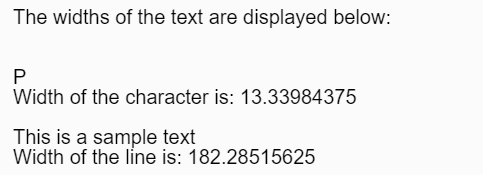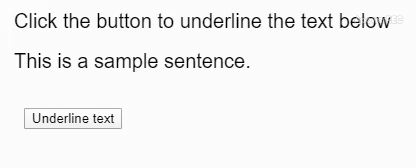Open in App
Not now

# p5.js | textWidth() Function

• Last Updated : 13 Dec, 2022

The textWidth() function is used to calculate the width of the text given as parameter. Syntax:

`textWidth( theText )`

Parameters: This function accepts a single parameter as mentioned above and described below:

• theText: It holds the string of which the width has to be measured.

Return Value: It returns a number which denotes the width of the given text. Below examples illustrate the textWidth() function in p5.js: Example 1:

## javascript

 `let sampleChar = "P";` `let sampleLine = "This is a sample text";` ` `  `// Canvas area creating` `function` `setup() {` `  ``createCanvas(400, 200);` `  ``textSize(20);` `  ``text(``'The widths of the text are '` `        ``+ ``'displayed below:'``, 20, 20);` `   `  `  ``// Checking textwidth sampleChar` `  ``text(sampleChar, 20, 80);` `  ``let charWidth = textWidth(sampleChar);` `  ``text("Width of the character is: " ` `        ``+ charWidth, 20, 100);` `   `  `  ``// Checking textwidth sampleLine ` `  ``text(sampleLine, 20, 140);` `  ``let lineWidth = textWidth(sampleLine);` `  ``text("Width of the line is: "` `        ``+ lineWidth, 20, 160);` `}`

Output:Example 2: Using text width measure to underline the text.

## javascript

 `let sampleText = ` `    ``"This is a sample sentence.";` ` `  `// Canvas area creating` `function` `setup() {` `  ``createCanvas(400, 200);` `  ``textSize(20);` `  ``text(``'Click the button to underline'` `        ``+ ``' the text below'``, 20, 40);` `  ``text(sampleText, 20, 80);` `   `  `  ``// Creating button` `  ``btn = createButton("Underline text");` `  ``btn.position(30, 120);` `  ``btn.mousePressed(underlineText);` `}` ` `  `// Measuring text width and` `// creating underline` `function` `underlineText() {` `  ``let width = textWidth(sampleText);` `  ``line(20, 90, width + 20, 90);` `}`

Output:Online editor: https://editor.p5js.org/ Environment Setup: https://www.geeksforgeeks.org/p5-js-soundfile-object-installation-and-methods/ Reference: https://p5js.org/reference/#/p5/textWidth

My Personal Notes arrow_drop_up
Related Articles